Get here NCERT Solutions Class 10 Maths 2. These NCERT Solutions for Class 10 of Maths subject includes detailed answers of all the questions in Chapter 2 – Polynomials provided in NCERT Book which is prescribed for class 10 in schools.

Resource: National Council of Educational Research and Training (NCERT) Solutions
Class: 10th Class
Subject: Maths
Chapter: Chapter 2 – Polynomials

NCERT Solutions Class 10 Maths Chapter 2 – Polynomials

NCERT Solutions for Class 10 maths chapter 2 Polynomials is available here. It includes solutions to all the questions present in class 10 Maths NCERT textbooks. These solutions are prepared by our subject experts as per the guidelines of NCERT, to help students in their academics.

What are Polynomials?

Polynomials are expressions which are composed of two algebraic terms. Students preparing for 10th Class board exams can make use of solved questions from these maths ncert solutions class 10, which contains important questions of Polynomial chapter.

NCERT Solutions Class 10 Maths Chapter 2 Polynomials Important Topics:

NCERT Solutions for class 10 maths Polynomials chapter 2 includes the major topics such as:

• Introduction to Polynomials
• Geometrical Meaning of the Zeros of Polynomial
• Relationship between Zeros and Coefficients of a Polynomial
• Division Algorithm for Polynomials

Polynomial class 10 notes is also available here with us for the students, to help them prepare for final exams. The major polynomials formulas are also explained in these solutions with solved questions. This is a great source of learning as it covers most of the syllabus as per given in NCERT books. Students can study topics of polynomial in different patterns with the help of exemplar problems of 10th Class 2nd chapter, to understand the concepts completely along with adequate knowledge on the subject. Students can download the Maths NCERT solutions for class 10 pdf notes, exercise-wise also.

Class 10th NCERT Solutions Maths Chapter 2 Polynomials Exercises

• NCERT Solutions Class 10 Maths Chapter 2 Polynomials Exercise 2.1
• NCERT Solutions Class 10 Maths Chapter 2 Polynomials Exercise 2.2
• NCERT Solutions Class 10 Maths Chapter 2 Polynomials Exercise 2.3

NCERT Solutions Class 10 Maths Chapter 2 Polynomials -DEGREE OF A POLYNOMIAL

If p(x) is a polynomial in x, the highest power of x in p(x) is called the degree of polynomial p(x).

And expressions like:- x2−−√+2,1x1etc., are not polynomials.

Example-1:

3x4+2x2+6x = 1, the highest power of the given polynomial equation is 4

Therefore, its degree is 4.

Example-2:

x5y3z + 2xy3+4x2yz2

This equation is of multiple variables (x, y, z) and to find the degree of this equation, we just need to add up the degrees of the variables in each of the terms and also it does not matter whether they are different variables.

Therefore, 1st term (5+3+1), 2nd term (1+3) and 3rd term (2+1+2).

The highest total is 9, of the 1st term,

Therefore, its degree is 9.

Polynomial equation with degree 1 is called a linear polynomial.

Example:     x – 3–√ = 0

Polynomial equation with degree 2 is called a quadratic polynomial.

Example:    x2+2–√x+7=.

Polynomial equation with degree 3 is called a cubic polynomial.

Example:    3x35x2+7–√x+5–√=.

Factorization of a quadratic polynomial equation by splitting middle term:-

General form of quadratic polynomial equation:- ax+ bx + c = 0

Step 1:- Find the product of a (first term) and c (last term).

Step 2:- Then split the product of a and c into two numbers such that their product remains same as (a × c) and their sum or difference is equal to the value of b (middle term).

Step 3:- Now, there will be total four terms in the equation, make two groups with each group having two terms each. Then take whatever is common and equate it to 0, the resultant values of x will be factors of the quadratic equation.

Example:

12 x2 = -11x +15

Solution:

12x 2 + 11x -15 = 0                (product of a and c is 12×15=180)

12x 2 + 20x – 9x -15 = 0      (splitting 180 into 20 and 9 such that, 20 – 9 = 11 and 20×9 = 180).

4x(3x + 5) – 3(3x + 5) = 0

(4x -3)(3x + 5) = 0

4x – 3 = 0 or 3x + 5 = 0

4x = 3 or 3x = – 5

x = 34 or x = 53

Therefore, solution of this quadratic equation  is (5334 ).

This solution is called zeroes or roots of the given polynomial.

NCERT Solutions Class 10 Maths Chapter 2 Polynomials EXERCISE 2.1

Graphical method to find zeroes:-

Total number of zeroes in any polynomial equation = total number of times the curve intersects x-axis.

Example 1:-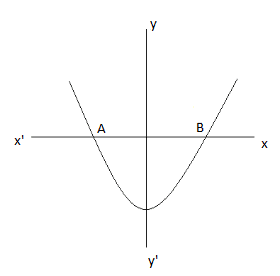In the above graph, the curve intersects x axis at A and B.

Therefore, the equation of the curve will have maximum of 2 zeroes.

Example 2:-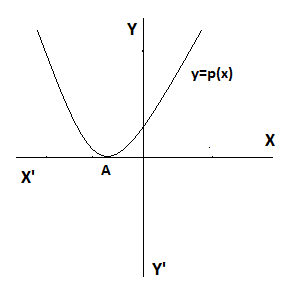From the above graph, the curve cuts the x-axis at point A

Therefore, number of zeroes of p(x) = 1

Example 3: –

From the above graph, the curve p(x) intersects x-axis at points AB and C.

Therefore, the number of zeroes of polynomial equation is 3.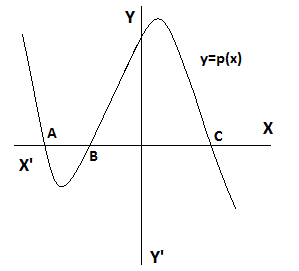Zeroes and Coefficient of a polynomial:

If α and β are zeroes of any quadratic polynomial ax2 + bx + c=0,

Then, Sum of zeroes = α+β = ba

Product of zeroes = αβ = ca

If α and β are zeroes of any quadratic polynomial, then the quadratic polynomial equation can be written directly as:

x2 – (α + β)x + αβ = 0

NCERT Solutions Class 10 Maths Chapter 2 Polynomials EXERCISE 2.2

Q.1 Find the zeroes of the following quadratic polynomials and verify the relationship between the zeroes and the coefficients.

(i) 6x² + 11x + 5 = 0

Soln: –

6x+ 11x + 5 = 6x+ 6x + 5x + 5

= 6x(x +1) + 5(x +1)

= (x +1) (6x +5)

∴ zeroes of polynomial equation 6x2 +11x +5 are { −1, 56 }

Now, Sum of zeroes of this given polynomial equation = −1+( 56 ) 116

But, the Sum of zeroes of any quadratic polynomial equation is given by = coeff.ofxcoeff.ofx2

= 116

And Product of these zeroes will be = 1×56 56

But, the Product of zeroes of any quadratic polynomial equation is given by = constanttermcoeff.ofx2

56

Hence the relationship is verified.

(ii) 4s2 – 4s + 1

Sol:

4s2 – 4s + 1 = 4s2 – 2s  2s + 1

= 2s (2s – 1) 1(2s – 1)

= (2s – 1) (2s – 1)

∴ zeroes of the given polynomial are: {12,12}

∴ Sum of these zeroes will be =  = 1.

But, The Sum of zeroes of any quadratic polynomial equation is given by = coeff.ofscoeff.ofs2

44 = 1

And the Product of these zeroes will be = 12×12 =14

But, Product of zeroes in any quadratic polynomial equation is given by = constanttermcoeff.ofs2

14.

Hence, the relationship is verified.

(iii) 6x2 – 3 – 7x

Sol:

6x2 – 7x – 3 = 6x2 – 9x + 2x – 3

= 3x (2x – 3) +1(2x – 3)

= (3x + 1) (2x – 3)

∴ zeroes of the given polynomial are: – (13,32)

∴ sum of these zeroes will be =  13+32 =76

But, The Sum of zeroes in any quadratic polynomial equation is given by = coeff.ofxcoeff.ofx2

76

And Product of these zeroes will be = 13×32=12 Also, the Product of zeroes in any quadratic polynomial equation is given by = constanttermcoeff.ofx2

36 = 12

Hence, the relationship is verified.

(iv) 4u2 + 8u

Sol:

4u2 + 8u = 4u (u+2)

Clearly, for finding the zeroes of the above quadratic polynomial equation either: – 4u=0 or u+2=0

Hence, the zeroes of the above polynomial equation will be (0, −2)

∴ Sum of these zeroes will be = −2

But, the Sum of the zeroes in any quadratic polynomial equation is given by = coeff.ofucoeff.ofu2

= 84 = −2

And product of these zeroes will be = 0 × −2 =

But, the product of zeroes in any quadratic polynomial equation is given by = constanttermcoeff.ofu2                                                                          = 4 = 0

Hence, the relationship is verified.

(v) t2 – 15

Sol:

t2 – 15 = (t+ 15−−√) (t − 15−−√)

Therefore, zeroes of the given polynomial are: – {15−−√, −15−−√}

∴ sum of these zeroes will be = 15−−√ −  15−−√ =

But, the Sum of zeroes in any quadratic polynomial equation is given by = coeff.ofxcoeff.ofx2

= 1 = 0

And the product of these zeroes will be = (15−−√) × (−15−−√)  = −15

But, the product of zeroes in any quadratic polynomial equation is given by

constanttermcoeff.oft2                                                                     = 151  = −15

Hence, the relationship is verified.

(vi) 3x2 – x – 4

Sol:

3x2 − x − 4   = 3x2 – 4x + 3x − 4

= x (3x – 4) +1(3x – 4)

= ( x + 1) (3x – 4)

∴ zeroes of the given polynomial are: – {−1, 43 }

∴ sum of these zeroes will be = −1 +43 = 13

But, the Sum of zeroes in any quadratic polynomial equation is given by = coeff.ofxcoeff.ofx2

(1)3 = 13

And the Product of these zeroes will be = {−1 × 43 }

43

But, the Product of zeroes in any quadratic polynomial equation is given by = constanttermcoeff.ofx2

43

Hence, the relationship is verified.

Q2. Form a quadratic polynomial each with the given numbers as the sum and product of its zeroes respectively.

(i). 26 , −3

Sol.

Given,

α + β = 26

αβ = −3

∴ If α and β are zeroes of any quadratic polynomial, then the quadratic polynomial equation can be written directly as:-

x2 – (α+β)x +αβ = 0

Thus, the required quadratic equation will be:

x2 – (26)x −3 = 0

6x− 2x – 18 = 0.

(ii). 3–√ , 43

Sol.

Given,

α + β = 3–√

αβ = 43

∴ If α and β are zeroes of any quadratic polynomial, then the quadratic polynomial equation can be written directly as: –

x2 – (α+β)x +αβ=0

Thus, the required quadratic equation will be: –

x2 – (3–√)x + 43 =0

3x− 33–√x + 4 = 0.

(iii).  0, 7–√

Sol.

Given,

α + β =

αβ = 7–√

∴ If α and β are zeroes of any quadratic polynomial, then the quadratic polynomial equation can be written directly as:-

x2 – (α+β)x +αβ=0

Thus, the required quadratic equation will be: –

x2 – (0)x + 7–√ = 0

x7–√ = 0.

(iv).  −2, −2

Sol.

Given,

α + β = −2

αβ = −2

∴ If α and β are zeroes of any quadratic polynomial, then the quadratic polynomial equation can be written directly as:

x2 – (α+β)x +αβ=0

∴ The required quadratic polynomial will be:

x2 – (−2)x −2 = 0

x+ 2x – 2 = 0.

(v). 7239

Sol.

Given,

α + β = 72

αβ = 39

∴ If α and β are zeroes of any quadratic polynomial, then the quadratic polynomial equation can be written directly as: –

x2 – (α+β)x +αβ = 0

∴ The required quadratic polynomial will be:

x2 – (72)x + 39 = 0

18x+ 63x + 6 = 0.

(vi). 6, 0

Sol.

Given,

α + β = 6

αβ =

∴ If α and β are zeroes of any quadratic polynomial, then the quadratic polynomial equation can be written directly as:-

x2 – (α+β)x +αβ = 0

∴ The required quadratic polynomial will be:-

x2 – 6x + 0 = 0

x2 – 6x = 0.

NCERT Solutions Class 10 Maths Chapter 2 Polynomials EXTRA QUESTIONS

Q.1 Find a quadratic polynomial whose zeroes are: –2+12212.

Sol.

Given: –

α + β = 2+12 + 212

= 4.

αβ = (2+12)(212)=412=72

∴ If α and β are zeroes of any quadratic polynomial, then the quadratic polynomial equation can be written directly as: –

x2 – (α+β)x +αβ = 0

Thus, the required quadratic polynomial equation will be :-

x2 – (4)x −72 = 0

2x2−8x+7 = 0.

Q.2 If α and β are the roots of a quadratic polynomial ax2+bx+c, then find the value of  α2 + β2.

Sol.

From the equation, (α+β=ba)

And,               α×β=ca

(α+β)2=α2+β2+(2αβ)

∴ α2+β2=(α+β)22αβ

∴ α2+β2=(ba)22ca

∴ α2+β2=b2a22ca

∴α2+β2=b22aca2.

Similarly, we can find out the values of (α3 + β3) and (α3 – β3).

Q3. If α and β are zeroes of a quadratic polynomial x2+4x+3, form the polynomial whose zeroes are  1+αβand1+βα.

Sol.

Since α and β are zeroes of a quadratic polynomial x2+4x+3,

α+β= -4, αβ = 3

Given: –  α1 = 1+αβ

β1βα

Now, sum of zeroes = 1+αβ+1+βα

2+αβ+βα

2αβ+α2+β2αβ

= (α+β)2αβ

On putting values of α+β and αβ from above we get:-

Sum of zeroes = α1 + β1 = 423

= 163

Now, Product of zeroes = (1+αβ)(1+βα)

=1+βα+αβ+αββα

=2αβ+β2+α2αβ

(α+β)2αβ

On putting values of α+β and αβ we get:

Product of zeroes = α1 × β1 = 423

163

Thus the required quadratic polynomial equation will be:-

x2 – (α1 + β1)x + α1β1 = 0

x2 – (163)x + 163 = 0

3x2 – 16x +16=0.

Q4. If α and β are zeroes of a quadratic polynomial p(x) = rx2+4x+4, Find the values of “r” if: – α2 + β2 = 24.

Sol.

From the given polynomial p(x),

α + β 4r, and αβ = 4r………. (1)

Since,(α + β)2 = α2 + β2 + 2αβ

Given,   α2 + β2 = 24 and from equation (1).

Therefore,  (4r)2=24+2×4r 16r2=24+2×4r

16 = 24 r+ 8r

3r+ r – 2 = 0

3r+ 3r – 2r -2 = 0

3r(r+1) – 2(r+1) = 0

(3r-2) (r+1) = 0

∴  r = 23   or   r = -1

NCERT Solutions Class 10 Maths Chapter 2 Polynomials DIVISION ALGORITHM

If suppose p(x) and g(x) are any two polynomials with g(x) ≠ 0, then we can find polynomials q(x) and r(x) such that: –

p(x)=g(x) × q(x) + r(x), where r(x)=0 or  degree of r(x) < degree of g(x) …….(1)

This is known as the division algorithm for polynomials.

And since, Dividend = Divisor × Quotient + Remainder

On comparing it with equation (1) we can conclude that:

Dividend = p(x).

Divisor = g(x).

Quotient = q(x).

Remainder = r(x).

NCERT Solutions Class 10 Maths Chapter 2 Polynomials EXERCISE 2.3

Q.1 If a polynomial x-3x2+x+2 is divided by a polynomial g(x), the quotient and remainder obtained are (x-2) and (-2x+4), respectively. Find the equation of g(x).

Sol.

Since, Dividend = Divisor × Quotient + Remainder

Therefore, x³ -3x2+x+2 = g(x) × (x-2) + (-2x+4)

(x-3x2+x+2) – (-2x+4) = g(x) × (x-2)

Therefore, g(x) × (x-2) = x-3x2+3x-2

Now, for finding g(x) we will divide “x-3x2+3x-2” with (x-2)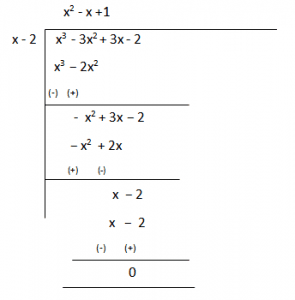Therefore, g(x) = (x2 – x +1)

Q.2 Find the quotient and remainder by dividing the polynomial f(x) by the polynomial g(x).

(i) f(x) = x+ 2x– 9x + 5,   g(x) = x2+5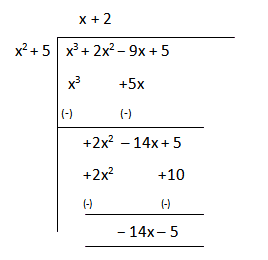Therefore, Quotient is (x+2) and Remainder is (-14x − 5)

(ii) f(x) = x5+2x4-9x3+5x2-2x+1, g(x) = x+ x– x+1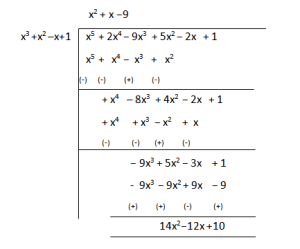Therefore, Quotient is (x+ x − 9) and Remainder is (14x2 – 12x +10).

(iii) f(x) = 2x+ 7x+ 5x+ 8x + 5, g(x) = 11− 2x+ x2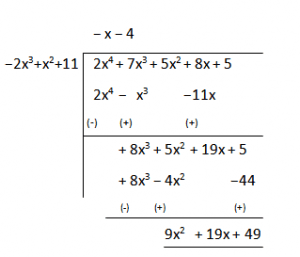Therefore, Quotient is  – (x + 4) and Remainder is (9x2 + 19x +49).

Q3. Find all the zeroes of the polynomial equation 2x4-3x3-3x2+6x-2, if two of its zeroes are 2–√and2–√.

Sol.

Since this is a polynomial equation of degree 4, there will be a total of 4 roots.

Let, f(x) = 2x– 3x– 3x+ 6x – 2

2–√and2–√ are zeroes of f(x).

(x2–√)(x+2–√)(x2− 2) = g(x), is a factor of given polynomial f(x).

If we divide f(x) by g(x), the quotient will also be a factor of f(x) with remainder =0.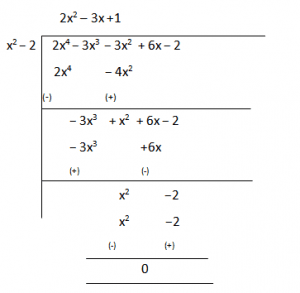So, 2x− 3x− 3x+ 6x – 2 = (x– 2) (2x2 – 3x +1).

Now, on further factorizing (2x2 – 3x +1) we get,

2x2 – 3x +1 = 2x2 – 2x − x +1 = 0

2x (x − 1) – 1(x−1) = 0

(2x−1) (x−1) = 0

So, its zeroes are given by:  x= 12 and x = 1

Therefore, all four zeroes of the given polynomial equation are:

2–√,2–√,12and1.

Q4. Find all zeroes of a polynomial equation x4-6x3-26x2+138x-35, if two of its zeroes are 2+3–√ and 23–√.

Sol.

Since this is a polynomial equation of degree 4, hence there will be total 4 roots.

Let, f(x) = x– 6x– 26x2+ 138x – 35

Since, (2+3–√)and(23–√) are zeroes of given polynomial f(x).

[x(2+3–√)][x(23–√)]=

(x23–√)(x2+3–√)

On multiplying the above equation we get,

x−4x + 1, this is a factor of a given polynomial f(x).

Now, if we will divide f(x) by g(x), the quotient will also be a factor of f(x) and the remainder will be 0.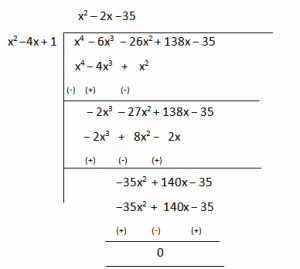So, x− 6x3  − 26x+ 138x – 35 = (x– 4x + 1) (x2 – 2x −35).

Now, on further factorizing (x2 – 2x −35) we get,

x2 – (7−5)x − 35 = x2 – 7x + 5x +35 = 0

x(x − 7) + 5 (x−7) = 0

(x+5) (x−7) = 0

So, its zeroes are given by:              x= −5 and x = 7.

Therefore, all four zeroes of given polynomial equation are:

2+3–√,23–√, −5 and 7.

Q5. Find all zeroes of a polynomial equation 3x+ 6x– 2x– 10x – 5, if two of its zeroes are 53−−√ and 53−−√.

Sol.

Since this is a polynomial equation of degree 4, hence there will be total 4 roots.

Let, f(x)= 3x4+6x3-2x2-10x-5

53−−√ and 53−−√ are zeroes of polynomial f(x).

(x53−−√)(x+53−−√)

(x253)=

(3x2−5)=0, is a factor of given polynomial f(x).

Now, when we will divide f(x) by (3x2−5) the quotient obtained will also be a factor of f(x) and the remainder will be 0.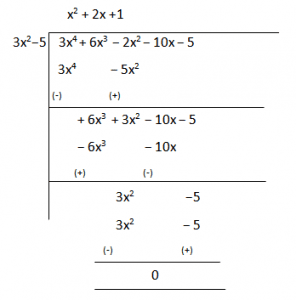Therefore, 3x+ 6x− 2x− 10x – 5 = (3x– 5) (x2 + 2x +1).

Now, on further factorizing (x2 + 2x +1) we get,

x2 + 2x +1 = x2 + x + x +1 = 0

x(x + 1) + 1(x+1) = 0

(x+1) (x+1) = 0

So, its zeroes are given by: x= −1 and x = −1

Therefore, all four zeroes of given polynomial equation are:

53−−√53−−√, −1 and −1.

NCERT Chapter 2, polynomials, is an important chapter for 10th standard board exams, which is going to be conducted this year, 2019. The marks scored in class 10th, will help you to move to your next class with your preferred subject or choice of subject. Also, with the help of Class 10th scorecard, you become qualified for getting jobs both in private and public sectors.